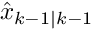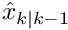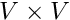Main MRPT website > C++ reference for MRPT 1.5.7
mrpt::utils::TMatchingPairList Class Reference

## Detailed Description

A list of TMatchingPair.

Definition at line 78 of file TMatchingPair.h.

#include <mrpt/utils/TMatchingPair.h>

Inheritance diagram for mrpt::utils::TMatchingPairList:## Public Member Functions

bool indexOtherMapHasCorrespondence (size_t idx) const
Checks if the given index from the "other" map appears in the list. More...

void dumpToFile (const std::string &fileName) const
Saves the correspondences to a text file. More...

void saveAsMATLABScript (const std::string &filName) const
Saves the correspondences as a MATLAB script which draws them. More...

float overallSquareError (const mrpt::poses::CPose2D &q) const
Computes the overall square error between the 2D points in the list of correspondences, given the 2D transformation "q"Whereare the elements of the square error vector as computed by computeSquareErrorVector. More...

float overallSquareErrorAndPoints (const mrpt::poses::CPose2D &q, std::vector< float > &xs, std::vector< float > &ys) const
Computes the overall square error between the 2D points in the list of correspondences, given the 2D transformation "q", and return the transformed points as well. More...

void squareErrorVector (const mrpt::poses::CPose2D &q, std::vector< float > &out_sqErrs) const
Returns a vector with the square error between each pair of correspondences in the list, given the 2D transformation "q" Each elementis the square distance between the "this" (global) point and the "other" (local) point transformed through "q":void squareErrorVector (const mrpt::poses::CPose2D &q, std::vector< float > &out_sqErrs, std::vector< float > &xs, std::vector< float > &ys) const
Returns a vector with the square error between each pair of correspondences in the list and the transformed "other" (local) points, given the 2D transformation "q" Each elementis the square distance between the "this" (global) point and the "other" (local) point transformed through "q":bool contains (const TMatchingPair &p) const
Test whether the given pair "p" is within the pairings. More...

void filterUniqueRobustPairs (const size_t num_elements_this_map, TMatchingPairList &out_filtered_list) const
Creates a filtered list of pairings with those ones which have a single correspondence which coincides in both directions, i.e. More...

## Public Attributes

elements
STL member. More...

## ◆ contains()

 bool TMatchingPairList::contains ( const TMatchingPair & p ) const

Test whether the given pair "p" is within the pairings.

Definition at line 143 of file TMatchingPair.cpp.

References mrpt::utils::begin(), and mrpt::utils::end().

## ◆ dumpToFile()

 void TMatchingPairList::dumpToFile ( const std::string & fileName ) const

Saves the correspondences to a text file.

Definition at line 29 of file TMatchingPair.cpp.

## ◆ filterUniqueRobustPairs()

 void TMatchingPairList::filterUniqueRobustPairs ( const size_t num_elements_this_map, TMatchingPairList & out_filtered_list ) const

Creates a filtered list of pairings with those ones which have a single correspondence which coincides in both directions, i.e.

the best pairing of element i in map this is the best match for element j in map other, and viceversa

Definition at line 204 of file TMatchingPair.cpp.

## ◆ indexOtherMapHasCorrespondence()

 bool TMatchingPairList::indexOtherMapHasCorrespondence ( size_t idx ) const

Checks if the given index from the "other" map appears in the list.

Definition at line 84 of file TMatchingPair.cpp.

References mrpt::utils::begin(), and mrpt::utils::end().

## ◆ overallSquareError()

 float TMatchingPairList::overallSquareError ( const mrpt::poses::CPose2D & q ) const

Computes the overall square error between the 2D points in the list of correspondences, given the 2D transformation "q"Whereare the elements of the square error vector as computed by computeSquareErrorVector.

squareErrorVector, overallSquareErrorAndPoints

Definition at line 120 of file TMatchingPair.cpp.

References mrpt::math::sum().

## ◆ overallSquareErrorAndPoints()

 float TMatchingPairList::overallSquareErrorAndPoints ( const mrpt::poses::CPose2D & q, std::vector< float > & xs, std::vector< float > & ys ) const

Computes the overall square error between the 2D points in the list of correspondences, given the 2D transformation "q", and return the transformed points as well.Whereare the elements of the square error vector as computed by computeSquareErrorVector

squareErrorVector

Definition at line 130 of file TMatchingPair.cpp.

References mrpt::math::sum().

## ◆ saveAsMATLABScript()

 void TMatchingPairList::saveAsMATLABScript ( const std::string & filName ) const

Saves the correspondences as a MATLAB script which draws them.

Definition at line 52 of file TMatchingPair.cpp.

## ◆ squareErrorVector() [1/2]

 void TMatchingPairList::squareErrorVector ( const mrpt::poses::CPose2D & q, std::vector< float > & out_sqErrs ) const

Returns a vector with the square error between each pair of correspondences in the list, given the 2D transformation "q" Each elementis the square distance between the "this" (global) point and the "other" (local) point transformed through "q":.

overallSquareError

Definition at line 154 of file TMatchingPair.cpp.

References mrpt::utils::begin(), mrpt::utils::end(), and mrpt::math::square().

Referenced by mrpt::slam::CICP::ICP_Method_LM().

## ◆ squareErrorVector() [2/2]

 void TMatchingPairList::squareErrorVector ( const mrpt::poses::CPose2D & q, std::vector< float > & out_sqErrs, std::vector< float > & xs, std::vector< float > & ys ) const

Returns a vector with the square error between each pair of correspondences in the list and the transformed "other" (local) points, given the 2D transformation "q" Each elementis the square distance between the "this" (global) point and the "other" (local) point transformed through "q":.

overallSquareError

Definition at line 177 of file TMatchingPair.cpp.

References mrpt::utils::begin(), mrpt::utils::end(), and mrpt::math::square().

## ◆ elements

 T std::vector< T >::elements
inherited

STL member.

 Page generated by Doxygen 1.8.14 for MRPT 1.5.7 Git: 8277875f6 Mon Jun 11 02:47:32 2018 +0200 at lun oct 28 01:50:49 CET 2019